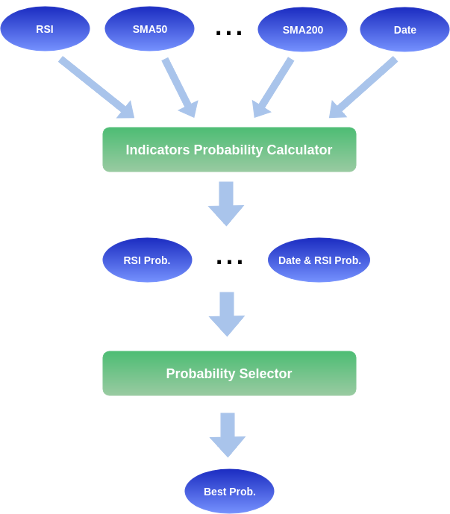## How Crystal Ball Works

#### Technology Introduction

Crystal Ball's core technology is based on our stock probability engine. For each individual stock's technical indicator combination, we compute the corresponding probability of stocks going up or down for different time frame. You can estimate probability of a stock going up or down for any given time frame from 1 trading day to more than 1000 trading days. The probabilities of each technical indicator pair can be found in the "Probability" page.

Since there are a lot of different probabilities, we created an algorithm that will pick the best probability. A list of best probabilities for different time frame can be found on the "Summary" page. The probability selection algorithm takes many factors into account. The algorithm is updated whenever we find ways to improve the prediction's probability.

Figure of How Crystal Ball Works#### What Should I Know About the Probabilities and Average Return?

1. What does a probability of P% mean?
It simply means that you have P% of generating 0% return or more.
2. What does an average return of R% mean?
It simply means that, if you invested many times under the same technical indicators, you would have an average return of R%. Seeing a 95% probability of going up with 40% average return does not guarantee that you will make money. If you did make money, the return could be lower than R%. One way to think about an average return of R% is that you have 50% chance for the return to be greater than or equal to R%.
3. What is the best way to use the probability?
Our probabilities works best when you select a bunch of high probability stocks into your portfolio. We recommend to have 10 positions or above. Typically, the higher the probability of the stock going up, the more weighting you want to give to the stocks. However, if you find it too troublesome, just use equal weighting (meaning you put the same amount of money in each stock).

When you select stocks, you also want to make sure your stocks are distributed among a few different sectors. There is a concept called sector rotation. This means that money would flow out from one sector to another. By having stocks in different sectors, you are ensured that your portfolio is not affected by sector rotation.
4. If I own 10 stocks and all them have at least 80% upside probability, am I guaranteed that 8 of my stocks will go up every time?
Unfortunately, no. There is no 100% that 8 of the stocks would go up. Under this circumstance, you can only consider what is the probability of 8 stocks will go up and 2 stocks will go down. You can estimate the probability of this situation occuring using the Binomial Distribution.

Furthermore, if the stock market or sector crashes, all of your high probability stocks can potentially go down. Stock market crashes are rare events. So, 20% chance of stock going down usually due to some really bad events like a stock market crash. When a market crashes, it will bring down lots of stocks with it. This is due to the fear of owning stocks and wanting to own pure cash. However, if you stick to 80% probability for the long term, you can be rest assured that 80% of the stocks you picked will go up.
5. So, even when I use probability to invest, I can lose money. How do I manage risk?
To manage risk, we recommend the use of the concept of risk-reward ratio. The idea is straightforward. If you predict that you have 50% chance of making \$1000, you should never risk losing more than \$1000 to generate the other \$1000 as a return. Otherwise, you will lose money if you repeat the process multiple times.

We see investing as a probabilistic activity. There are no investor that can make a return on all their investments. As we mentioned earlier, the average return can be thought of the return that you will generate 50% of the time. Therefore, if you can generate R%, you should never lose more than -R%. However, to make money in the long run, the total positive return must greater than the total negative return. This means that you want to lose -(R/N)% for every opportunity that would generate R%.

The number, N, is chosen by you. We recommend anywhere from 2 to 4. You need to understand that as N becomes larger, the more likely you will lose money due to price volatility. If you are new, you might want to choose N=2. As you become better at identifying bottoms in prices, you can increase N to 3 or 4.

To summarize, if you are new, then you should put a stop loss at half of the average return predicted by Crystal Ball. When the return does hit Crystal Ball's predicted average return, you might sell the stock or set tight stop loss while holding onto it.

Use our  "Watchlist"  feature to manage risk.